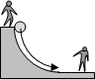# Canisters Rolling Down a Half Pipe

• onegreatweb

## Homework Statement

Oddjob rolls a canister of nerve gas twoards Bond, who is at the bottom of a curved trench. The canister has a mass of 16 kg, a radius of r = 0.8 m, and a rotational inertia of 10 kg*m2. It starts with no velocity. If the trench has a radius of R = 3.5 m, how fast is the canister moving when it reaches Bond?

## Homework Equations

Conservation of Energy: Ei = Ef

## The Attempt at a Solution

PEi = KEf + KErotation
mgR = (1/2)m*vf2 + (1/2)I*ω2
mgR = (1/2)m*vf2 + (1/2)I*(vf/R)2
mgR = vf2 (m/2 +I/(2R2))
vf2 = (2*m*g*R3) / (m*R2 + I)
vf = sqrt((2*m*g*R3) / (m*R2 + I))

I plugged in all the numbers but the computer says this is incorrect. Where did I go wrong?

Edit: I posted the wrong question initally. Oops. This is the correct one with the correct image.

#### Attachments

•bond1h.gif
1.9 KB · Views: 427
Last edited:
Is Bond at the bottom of the trench? Or on the opposite side?